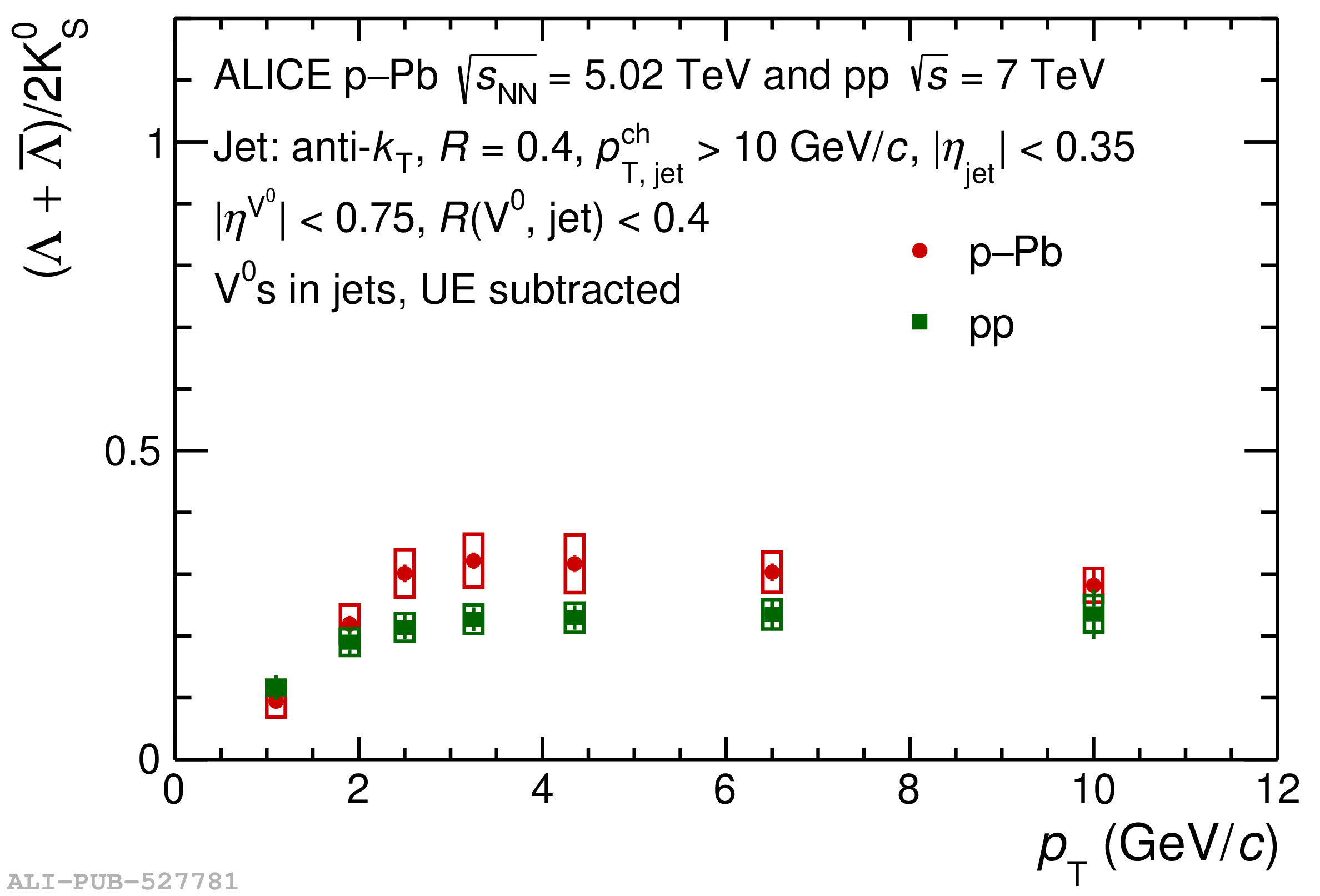# Figure 6

 The $\rLK$ ratio in pp collisions at \seven and in \pPb\ collisions at \fivenn as a function of \Vzero{}-particle $\pT$ associated with charged particle jets with $\pTjch>10$ \GeVc reconstructed using the \akT\ jet finder with resolution parameter $R=0.4$ The ratio is shown for the same selection of the matching radius $\Rvj<0.4$ in both systems The systematic uncertainties (open boxes) are uncorrelated between the systems.